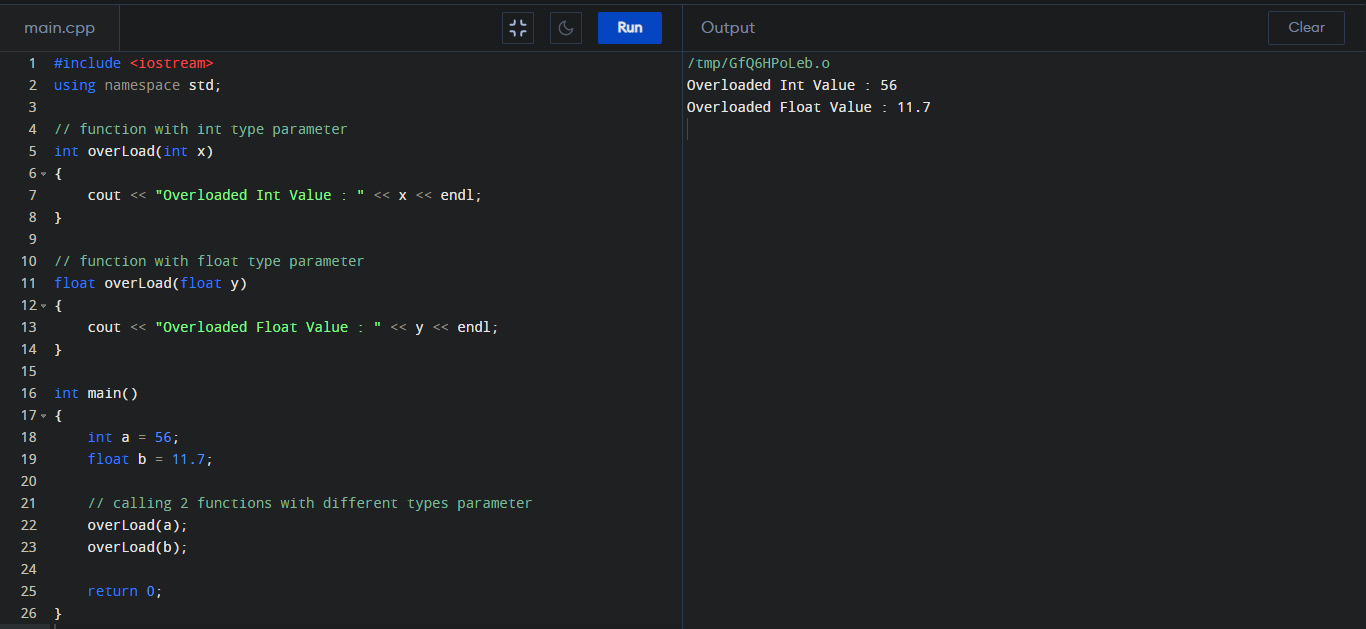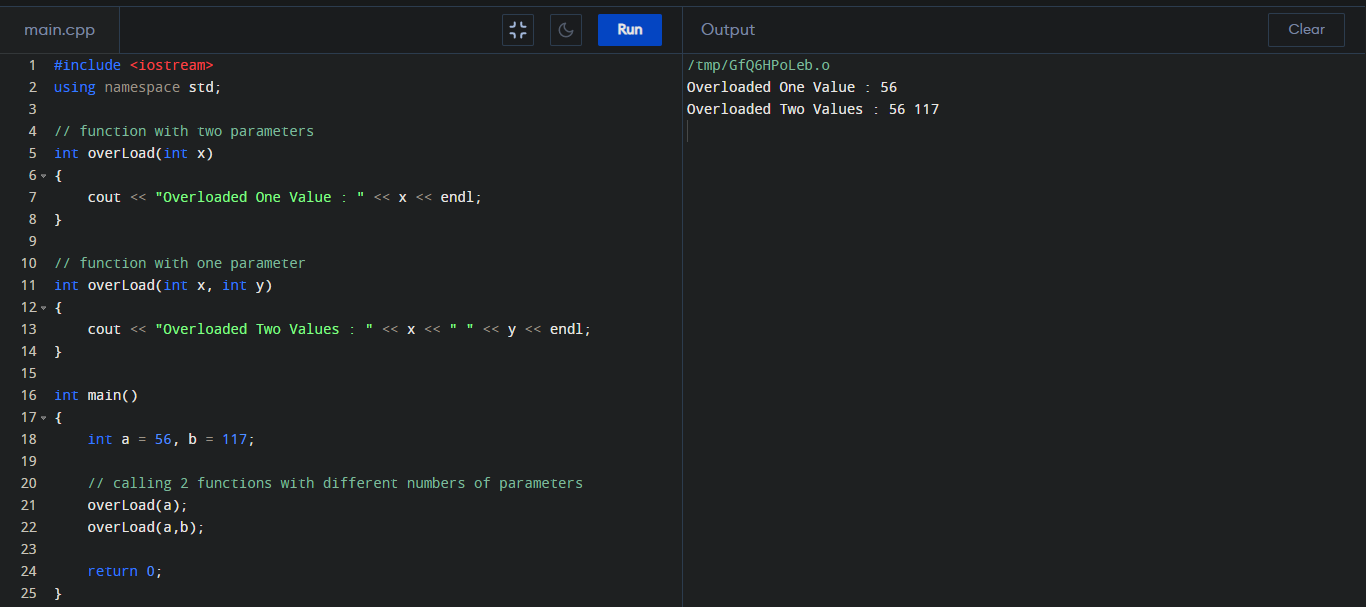Function overloading is a feature where two or more functions can have the same name if the number and/or type of arguments passed is different.

These functions having the same name but different arguments are known as overloaded functions.

Example :

//same name different arguments

int eruditors(int x) { }
float eruditors (float x) { }
int eruditors (int x, int y) { }

There are two types of functions in C++  :-

Program :

#include <iostream>
using namespace std;

// function with int type parameter
{
cout << "Overloaded Int Value : " << x << endl;
}

// function with float type parameter
{
cout << "Overloaded Float Value : " << y << endl;
}

int main()
{
int a = 56;
float b = 11.7;

// calling 2 functions with different types parameter

return 0;
}

Output :#include <iostream>
using namespace std;

// function with two parameters
{
cout << "Overloaded One Value : " << x << endl;
}

// function with one parameter
{
cout << "Overloaded Two Values : " << x << " " << y << endl;
}

int main()
{
int a = 56, b = 117;

// calling 2 functions with different numbers of parameters